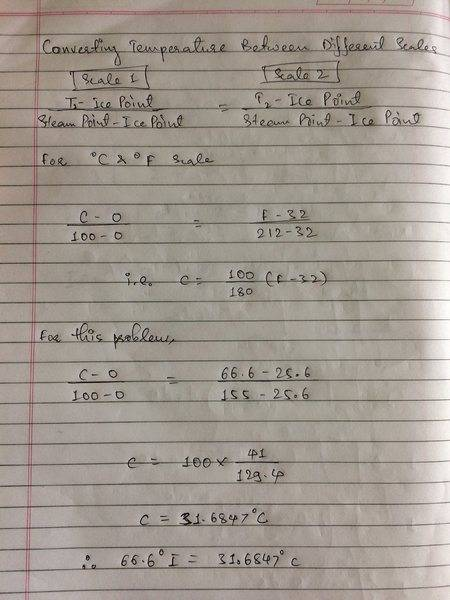# Converting between a known and unknown temperature scale

BOAS

## Homework Statement

On a Temperature scale, the ice point is 25.6°I and the steam point is 155°I. If the temperature reads 66.6°I what is the equivalent in °C?

## The Attempt at a Solution

25.6°I = 0°C

155°I = 100°C

1°I = 5/647 of the interval between boiling and freezing.

1C° = 647/500 I°

1I° = 500/647C°

66.6°I = 51.5°C

I arrive at this answer but i'm not convinced i've done this correctly. When changing from faranheit to celsius for example, I have to subtract the offset from zero...

So should my answer actually be 51.5 - 25.6 °C?

I'd really appreciate some help,

thanks!

Mentor
66.6°I = 51.5°C
That's not correct. And I don't understand where you get fractions like 5/647.

I think that an easier way to do the problem is to try to solve for the formula
$$a T_I + b = T_C,$$
where ##T_I## is the temperature in °I and ##T_C## is the temperature in °C. Using the data you have, that gives you two equations for two unknowns (##a## and ##b##).

Mentor
After recalculating a few things, I find that
1C° = 647/500 I°

1I° = 500/647C°
is correct. The problem is the shifting of the scale. Neither
66.6°I = 51.5°C
nor
So should my answer actually be 51.5 - 25.6 °C?
are correct.

Homework Helper
Note that Dr. Claude is using the fact that the relation between the two temperature scales is linear- one degree Celcius converts to the same number of degrees Farenheit, and vice-versa, at any temperature.

BOAS
That's not correct. And I don't understand where you get fractions like 5/647.

I think that an easier way to do the problem is to try to solve for the formula
$$a T_I + b = T_C,$$
where ##T_I## is the temperature in °I and ##T_C## is the temperature in °C. Using the data you have, that gives you two equations for two unknowns (##a## and ##b##).

When converting between faranheit and celsius, we say that 1°F = 1/180 of the interval between the boiling and freezing point.

Compared to the 1/100 of the interval that 1°C expresses.

9/5 of a degree faranheit is equivalent to 1 degrees celsius.

It was this method I was trying to use that gave me those strange fractions...

I haven't seen the method you are talking about before, please could you apply it to something so I can see what you mean?

Mentor
Note that Dr. Claude is using the fact that the relation between the two temperature scales is linear- one degree Celcius converts to the same number of degrees Farenheit, and vice-versa, at any temperature.
Right. It is implicit in the problem. Good of you for pointing this out.

BOAS
After recalculating a few things, I find that

is correct. The problem is the shifting of the scale. Neither

nor

are correct.

Ok,

I think this makes sense to me, I hope it does to you.

°C = ([°I] - 25.6) x 500/647

giving me the answer that 66.6°I = 31.7°C

Mentor
Edit: You got the correct answer in the previous post while I was typing the following. I'll leave it in case someone else has a similar problem.

I haven't seen the method you are talking about before, please could you apply it to something so I can see what you mean?
Lets say we want to rederive the conversion from Fahrenheit to Celcius. Take

freezing point: 32 °F = 0 °C
boiling point: 212 °F = 100 °C

We look for an equation of the type
$$a T_F + b = T_C$$
For the values above
$$a \times 32 + b = 0 \\ a \times 212 + b = 100$$
From the first equation, we have ##b = -32 a##. Using that in the second equation, we get
$$212 a - 32 a = 100 \Rightarrow a = \frac{100}{180} = \frac{5}{9}$$
and ##b = -160 / 9##. So
$$\frac{5}{9} T_F - \frac{160}{9} = T_C$$

I realize now that this is not the usual formula. You should therefore try it yourself using
$$\left( T_F + a \right) \times b = T_C$$
to get the factors you know. You can then do the same for your problem.

Mentor
Ok,

I think this makes sense to me, I hope it does to you.

°C = ([°I] - 25.6) x 500/647

giving me the answer that 66.6°I = 31.7°C
Correct!

•1 person
BOAS
Correct!

Thank you for the help

IamtheonewhoknocksThis is a detailed explanation on how to solve this problem and solve any problem based on temperature scale conversion. It's very easy. Hope it helps. I AM THE ONE WHO KNOCKS.